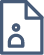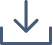### Nexus Between Randomised Numerical Linear Algebra and Big Time Series Data

A time series is a collection of random variables indexed according to the order in which they are observed in time. The main objective of time series analysis is to develop a statistical model to forecast the future behaviour of the system. A particular model is an Autoregressive Moving Average (ARMA) which is used for analysing stationary time series and a special case of this model is an Autoregressive (AR) model, which merely includes the autoregressive component.

In problems involving big time series data, selecting an appropriate AR model amounts to computing the solutions of many potentially large-scale Ordinary Least Squares (OLS) problem, which can be the main bottleneck of computations. Here is where randomised sub-sampling algorithms can be used to greatly speed-up such model selection procedures. For computations involving large matrices in general, and large-scale OLS problems in particular, randomised numerical linear algebra (RandNLA) has successfully employed various random sub-sampling and sketching strategies. There, the underlying data matrix is randomly, yet appropriately, compressed into a smaller one, while approximately retaining many of its original properties. As a result, much of the expensive computations can be performed on the smaller matrix.

In this research project we look to extend an already existing RandNLA algorithm, used for fitting appropriate AR models based on big time series data, to an algorithm to fit a more general ARMA model. However, unlike the AR model, the likelihood function in ARMA would be a non-convex nonlinear function which makes the problem more complicated. Perhaps, one approach, is utilising the Hannan-Rissanen algorithm which allows MA models to be approximated using high-order AR models. Here, we aim to develop a new algorithm with a nexus between these two algorithms, and evaluate its performance on several large-scale synthetic and real big time series data.#### Thomas McCarthy McCann

The University of Newcastle

Thomas is an undergraduate student in the School of Mathematical and Physical Sciences at the University of Newcastle where he studies a Bachelor of Mathematics majoring in Statistics. Currently in his third and final year of undergraduate study, Thomas enjoys the practicality of mathematics and statistics in solving real-world problems.

At the conclusion of his undergraduate degree, Thomas plans to continue his studies in an Honours year and sees the AMSI Vacation Research Scholarship as a perfect segue into this.

Outside of university, his other interests include sport, music and sneakers.